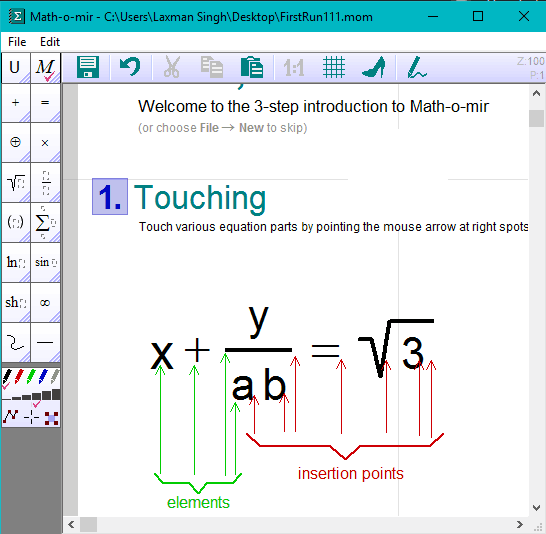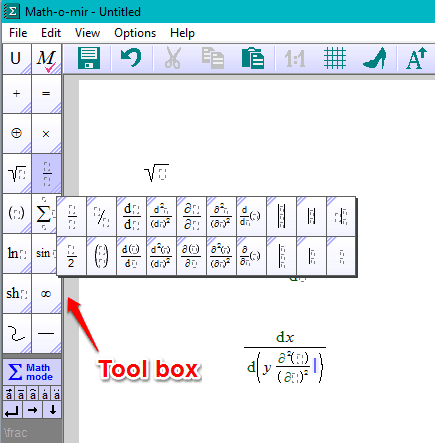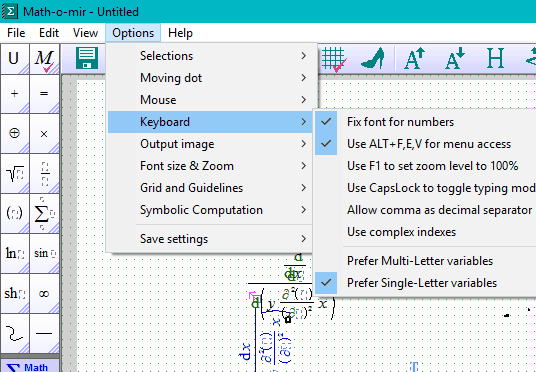Editor Ratings:
User Ratings:
[Total: 4 Average: 3]

Math-o-mir is a free software to write and edit math equations. It includes dozens of tools, supports hotkeys, and mouse cursor to insert algebra equations, derivatives, integrations, square roots and other mathematical operations easily. It is built to write math equations as easy as writing them in paper using a pencil. Also, it gives a quick shortcut to take image of equation and copy to MS Word.

Apart from above features, the software also has some other tools, like selection, arrow, eraser pencil, ellipse, and more. Your math equations can also be saved to PC for later use. So, overall, it is a good digital notepad for writing and editing math equations. However, the equations can be saved in its native format (MOM file) only. Still, I believe the software is good enough to practice and solve equations or math questions.You may also try any of these free Math equation editor extensions for Google Chrome.

## Using This Free Math Equation Editor Software:

• you can copy an equation element using left mouse button
• you can also copy the equation image (F9 hotkey) and paste it in MS Word or some other document editor
• hold down the ‘Alt‘ key to enter an exponent
• start typing on an empty area by clicking the left mouse button, etcIt won’t take much time to learn the basics. After this, you can start with a new file to write the math equations. The tools available at the left side will help you enter the integers, sigma, square roots, differentiation, and other operations.To select vertical text orientation, eraser pencil, ellipse, and other tools, you can use the upper middle section of its interface.

There are few more options you can use to work with this software comfortably. For example, you can set font size & zoom level, enable preset hotkey to access File menu, make selection bold, and more. The Options menu has all these features.Use the features as per your need and solve math equations.

When you have done practicing or editing the equations, you can save your work.

## Conclusion:

Math-o-mir is surely a good option for students, teachers, as well as engineers to write and edit math equations. The are plenty tools to insert math equations and other tools to explain or illustrate the equations.